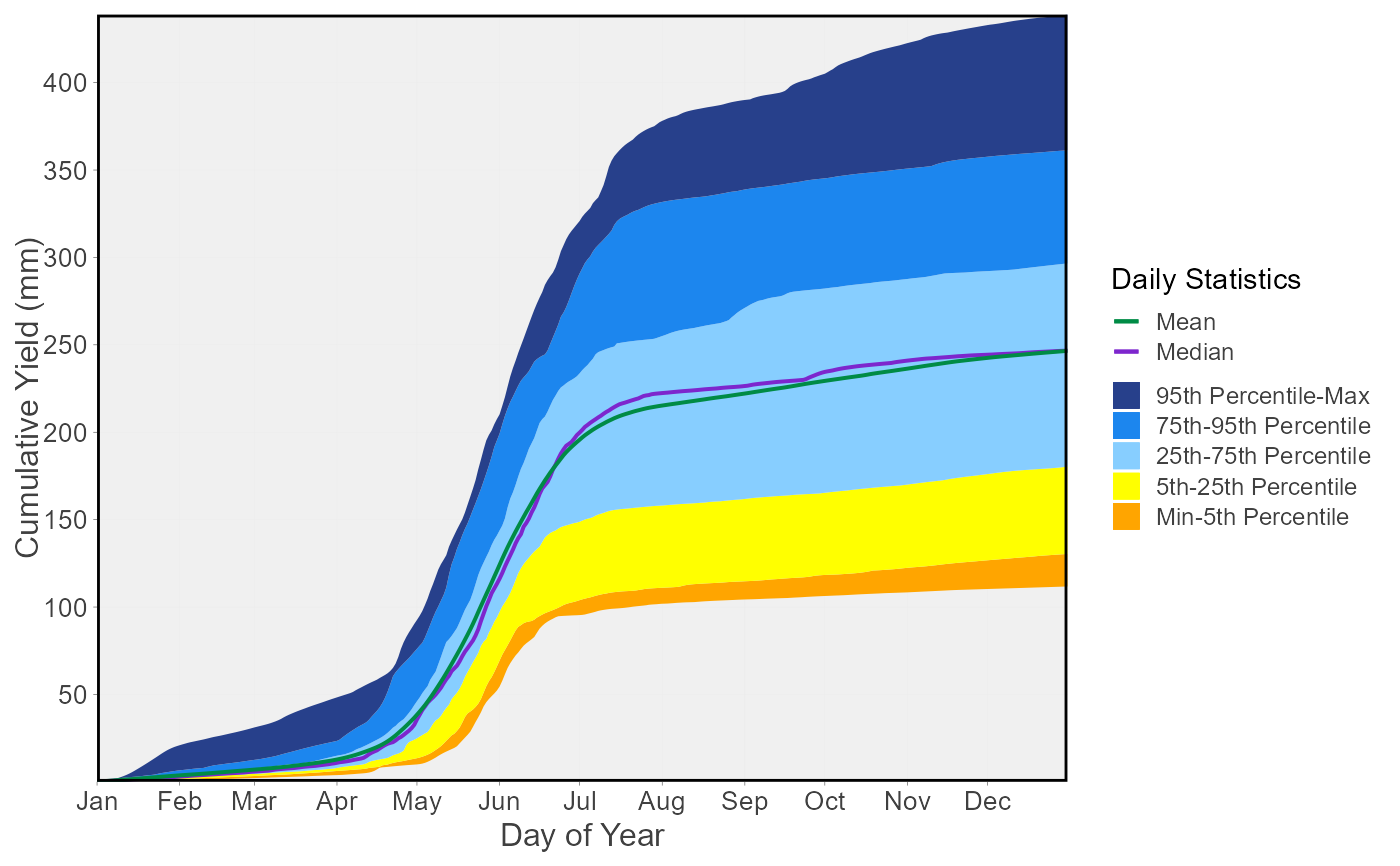Plot the daily cumulative mean, median, maximum, minimum, and 5, 25, 75, 95th percentiles for each day of the year from a daily streamflow data set. Calculates statistics from all values from complete, unless specified. Data calculated using calc_daily_cumulative_stats() function. Can plot individual years for comparison using the add_year argument. Defaults to volumetric cumulative flows, can use use_yield and basin_area to convert to water yield. Returns a list of plots.

plot_daily_cumulative_stats(
data,
dates = Date,
values = Value,
groups = STATION_NUMBER,
station_number,
use_yield = FALSE,
basin_area,
water_year_start = 1,
start_year,
end_year,
exclude_years,
log_discharge = FALSE,
include_title = FALSE,
add_year
)

## Arguments

data Data frame of daily data that contains columns of dates, flow values, and (optional) groups (e.g. station numbers). Leave blank if using station_number argument. Name of column in data that contains dates formatted YYYY-MM-DD. Only required if dates column name is not 'Date' (default). Leave blank if using station_number argument. Name of column in data that contains numeric flow values, in units of cubic metres per second. Only required if values column name is not 'Value' (default). Leave blank if using station_number argument. Name of column in data that contains unique identifiers for different data sets, if applicable. Only required if groups column name is not 'STATION_NUMBER'. Function will automatically group by a column named 'STATION_NUMBER' if present. Remove the 'STATION_NUMBER' column beforehand to remove this grouping. Leave blank if using station_number argument. Character string vector of seven digit Water Survey of Canada station numbers (e.g. "08NM116") of which to extract daily streamflow data from a HYDAT database. Requires tidyhydat package and a HYDAT database. Leave blank if using data argument. Logical value indicating whether to calculate area-based water yield, in mm, instead of volumetric discharge. Default FALSE. Upstream drainage basin area, in square kilometres, to apply to observations. Three options: (1) Leave blank if groups is STATION_NUMBER with HYDAT station numbers to extract basin areas from HYDAT. (2) A single numeric value to apply to all observations. (3) List each basin area for each group/station in groups (can override HYDAT value if listed) as such c("08NM116" = 795, "08NM242" = 10). If group is not listed the HYDAT area will be applied if it exists, otherwise it will be NA. Numeric value indicating the month (1 through 12) of the start of water year for analysis. Default 1. Numeric value of the first year to consider for analysis. Leave blank to use the first year of the source data. Numeric value of the last year to consider for analysis. Leave blank to use the last year of the source data. Numeric vector of years to exclude from analysis. Leave blank to include all years. Logical value to indicate plotting the discharge axis (Y-axis) on a logarithmic scale. Default FALSE. Logical value to indicate adding the group/station number to the plot, if provided. Default FALSE. Numeric value indicating a year of daily flows to add to the daily statistics plot. Leave blank for no years.

## Value

A list of ggplot2 objects with the following for each station provided:

Daily_Cumulative_Stats

a plot that contains daily cumulative flow statistics

Default plots on each object:
Mean

daily cumulative mean

Median

daily cumulative median

Min-5 Percentile Range

a ribbon showing the range of data between the daily cumulative minimum and 5th percentile

5-25 Percentiles Range

a ribbon showing the range of data between the daily cumulative 5th and 25th percentiles

25-75 Percentiles Range

a ribbon showing the range of data between the daily cumulative 25th and 75th percentiles

75-95 Percentiles Range

a ribbon showing the range of data between the daily cumulative 75th and 95th percentiles

95 Percentile-Max Range

a ribbon showing the range of data between the daily cumulative 95th percentile and the maximum

'Year' Flows

(optional) the daily cumulative flows for the designated year

## See also

calc_daily_cumulative_stats

## Examples

# Run if HYDAT database has been downloaded (using tidyhydat::download_hydat())
if (file.exists(tidyhydat::hy_downloaded_db())) {

# Plot annual daily volume statistics
plot_daily_cumulative_stats(station_number = "08NM116")

# Plot annual daily yield statistics with default HYDAT basin area
plot_daily_cumulative_stats(station_number = "08NM116",
use_yield = TRUE)

# Plot annual daily yield statistics with custom basin area
plot_daily_cumulative_stats(station_number = "08NM116",
use_yield = TRUE,
basin_area = 800)

}
#> Warning: One or more years contained partial data and were excluded. Only years with complete data were used for calculations.#> Warning: One or more years contained partial data and were excluded. Only years with complete data were used for calculations.#> Warning: One or more years contained partial data and were excluded. Only years with complete data were used for calculations.#> \$Daily_Cumulative_Yield_Stats#>